Forgot your password? User-login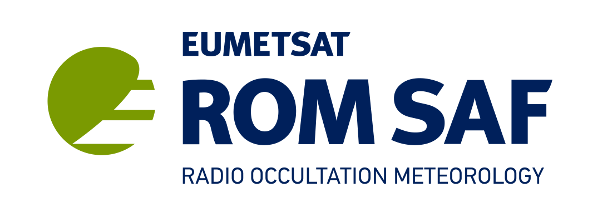|||
 About Home RO method News Archive Contact Search Documentation Product Documents Publications ROM SAF Reports Visiting Scientist User Workshops Data & Software Product Archive Product Quality Browse Occultations NRT Monitoring Climate Monitoring Software User Service Helpdesk Helpdesk History RSS Feeds Registration Login
 Software Error covariance

# Error Covariance Matrices

The error covariance matrix (ECM) is a dataset that specifies the correlations in the observation errors between all possible pairs of vertical levels. It is given as a two-dimensional array, of size NxN , where N is the number of vertical levels in the sounding data products.

Specifically, the ECM for variable X is given by E[(X - E[X])(X - E[X])T], where in practice the expectation value is estimated by an average over a representative sample.  It is a matrix because levels are treated independently: X is a vector of length equal to the number of vertical levels.

X could be an observation (refractivity N or bending angle α), in which case the ECM is sometimes called O, or a background state ({T, q, p*} for ECMWF-like models; {p, q} for Met Office-like models), in which case the ECM is sometimes called B. Both need to be specified in the minimisation of the usual 1dvar cost function

J(x) = ½(x-b)TB-1(x-b) + ½(H(x)-y)TO-1(H(x)-y))

which is needed to produce a retrieved analysis x.

ECMs are positive semi-definite matrices which can be factorised as

ECM = DT C D

where the diagonal matrix D comprises the standard deviations of X, and defines the "size" of the variance, while the correlation matrix C describes the "shape" of the variance of X. By definition of standard deviation, the diagonal terms of C are all equal to 1.

The ROPP user has a choice of whether to use fixed standard deviations D, read from an auxiliary covariance file, or to allow them to vary profile-by-profile, by including them in the observation or background file. At present, correlations C can only be read from the same auxiliary file. It is the content of these auxiliary covariance files which is described here.

In ROPP, the correlations in the covariance files are stored in packed, "triangular" format, so that successive elements of the variable "corr" hold the following matrix elements:

 1 2 4 7 ... 3 5 8 ... 6 9 ... 10 ... ...

By exploiting the symmetry of the correlation coefficients, only ½N(N+1) of them need to be stored.

The correlation matrices can be binned (in the same file) according to latitude. In principle this could be extended to seasonality. Standard deviations can also be held in the same file.  These, rather than the profile-by-profile SDs, will be used in the retrieval if  bg/obs_covar_method = FSFC or FSDC.

Further details on all the above can be found in the ROPP FM and 1DVAR User Guide.

Below, there are error-covariance matrices for the following:
• Covariance matrices for NRT products
• Covariance matrices for Reprocessed climate data records
• Other covariance matrices (included in ROPP)
Each matrix can be provided in several versions reflecting variations with geographical areas (e.g. latitude) and with season.

## Covariance matrices for NRT products

 Description Image (click to expand) File Background Suitable for 137L ECMWF background state. Currently, the T and p* standard deviations are the ones shown in the file.  The standard deviation of q is calculated profile-by-profile, using the background q multiplied by a fixed .  These standard deviations are then used to calculate the covariances using the given correlation matrix as ECM = D C D. The block diagonal structure of correlation matrix arises from use of a 3 element state vector: {T, q, p*}.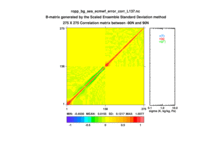ropp_bg_ecmwf_error_corr_L60.nc ropp_bg_ses_ecmwf_error_corr_L137.nc Observations Suitable for 247L refractivity retrievals. (Bending angle correlation matrices are usually assumed to be diagonal.) Note that there is a minimum σ(N) of 0.02 N-units above about 25km.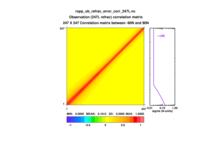ropp_ob_refrac_error_corr_247L.nc

## Covariance matrices for Reprocessed climate data records

 Description Image (click to expand) File Background Suitable for 60 L ERA-I background profiles. Average of ERA-I error of first guess resolved on 5 deg latitude intervals. Pressure standard deviation has been inflated to compensate for bias of ERA-I forecast with respect to ERA-I analysis.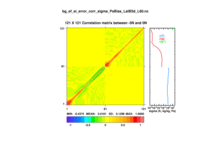ropp_bg_ecmwf_error_corr_L60.nc bg_ef_ei_error_corr_sigma_PsBias_LatB5d_L60.nc

## Other covariance matrices (included in ROPP)

Some example ECMs are available in the ropp_1dvar/errors directory of the ROPP distribution, for the benefit of the users. They are described below.

 Description Image (click to expand) File Background Suitable for (early) 60L ECMWF background state {T, q, p*}. Note that correlation matrix assumes level 1 at the top of the atmosphere.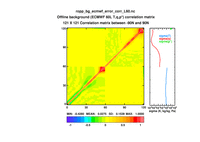ropp_bg_ecmwf_error_corr_L60.nc Background Suitable for (current) 91L ECMWF background state {T, q, p*}. Note that correlation matrix assumes level 1 at the bottom of the atmosphere.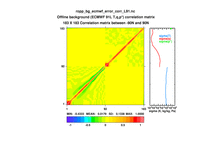ropp_bg_ecmwf_error_corr_L91.nc Background Suitable for (early) 50L Met Office background state {p, q}. Three latitude bands: -90 to -20, -20 to 20, 20 to 90N.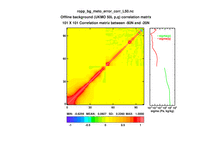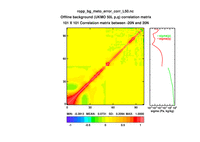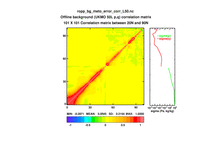ropp_bg_meto_error_corr_L50.nc Background Suitable for (current) 70L Met Office background state {p, q}. Three latitude bands: -90 to -20, -20 to 20, 20 to 90N.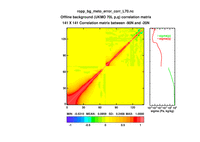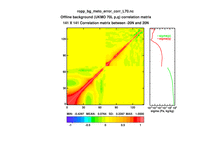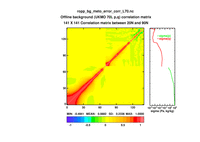ropp_bg_meto_error_corr_L70.nc Observation Suitable for 300L bending angles. (Note that the correlation matrix C is the identity matrix).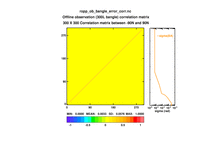ropp_ob_bangle_error_corr.nc Observation Suitable for standard 247L refractivities.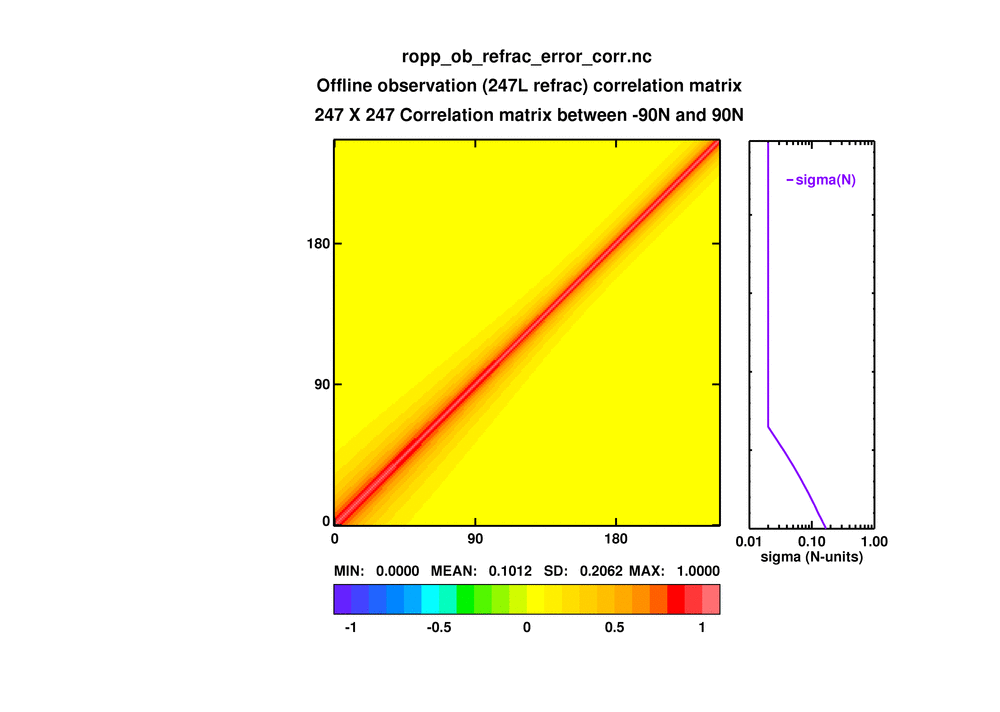ropp_ob_refrac_error_corr.nc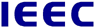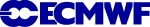Copyright © 1999-2023 EUMETSAT